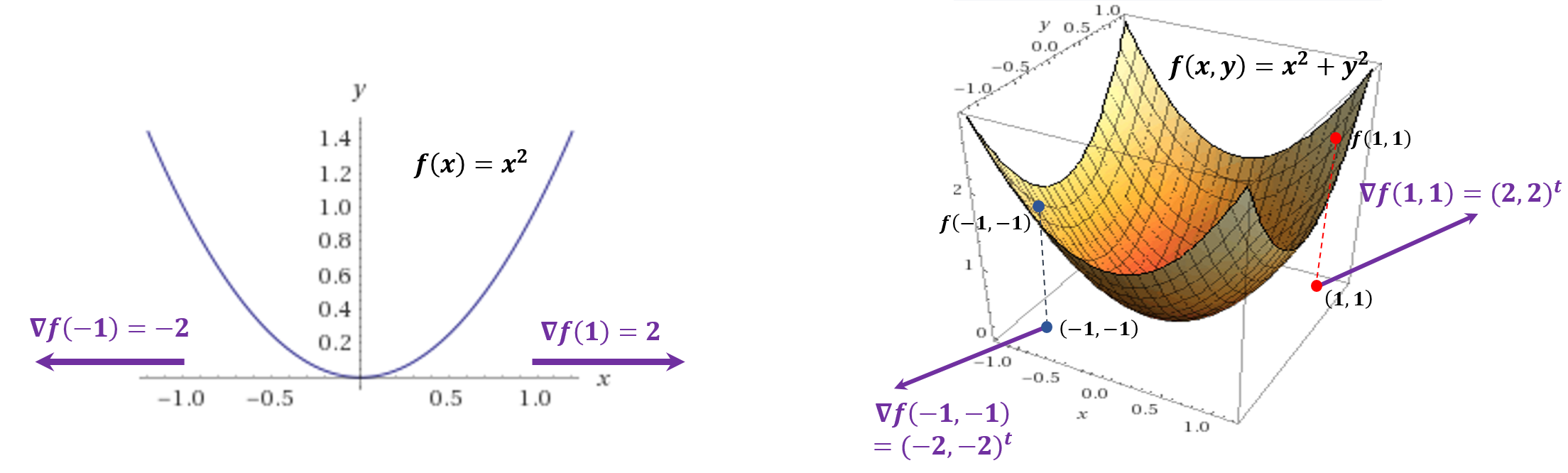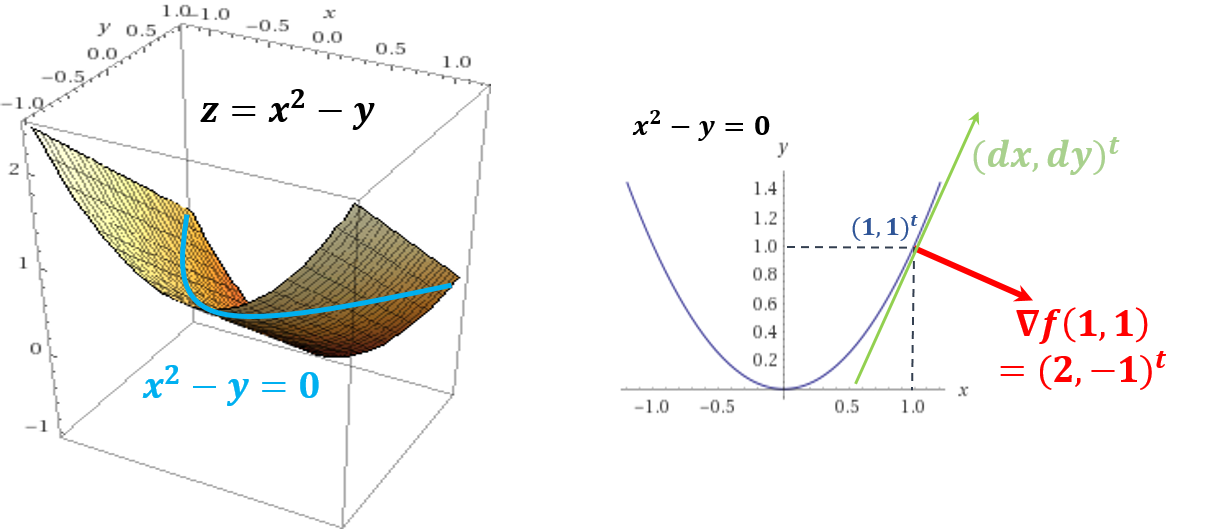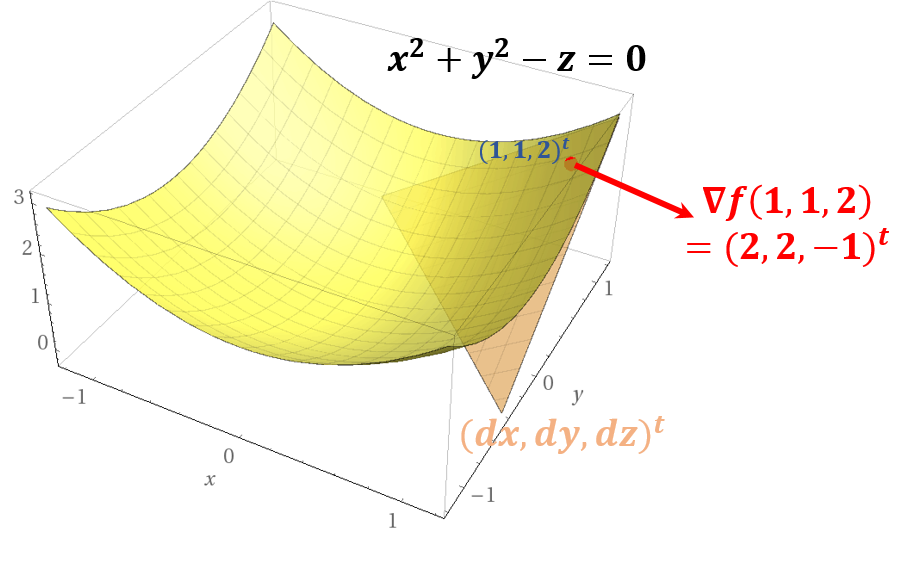The gradient of a function $f$ is $\nabla f: \R^n \to \R$, \begin{aligned} \nabla f(x) = \left(\begin{array}{c} \frac{\partial f(x)}{\partial x_1} \\ \vdots \\ \frac{\partial f(x)}{\partial x_n} \end{array}\right) \end{aligned}

## 1. The gradient points to direction $f$ is increasing.

By Taylor Theorem, for $s$ near $x$, \begin{aligned} f(x + s) \approx f(x) + \nabla f(x)^t s \end{aligned}

For maximizing $f$, we can choose a good $s$, which means $x$ should be moved to the direction $f$ is increasing. Note that $\nabla f(x)^t s$ is maximized when $f$ is maximized. As $\nabla f(x)^t s$ is the inner product of two vectors. \begin{aligned} \nabla f(x)^t s = \left\| \nabla f(x) \right\| \left\| s \right\| \cos\theta \end{aligned}

where $\theta$ is the angle between $\nabla f(x)$ and $s$. It is maximized when $\theta = 0$. In other words, when $\nabla f(x)$ and $s$ have the same direction, it is maximized. Therefore, $x$ should be moved to $\nabla f(x)$ direction to locally maximized $f$. For example, consider $f(x) = x^2$ and $f(x, y) = x^2 + y^2$ for $x$, $y \in \mathbb{R}$. Then their gradients are $\nabla f(x) = 2x$ and $\nabla f(x, y) = (2x, 2y)^t$.Their gradient point to the direction each $f$ is increasing at the point $x$. Moreover, $-\nabla f(x)$ points to the direction $f$ is decreasing.

## 2. The gradient is perpendicular to the tangent plane in terms of an implicit function.

The gradient has the different meaning for explicit and implicit functions

• The gradient of an explicit function $y = f(x)$ means the tangent vector at $x$.
• The gradient of an implicit function $f(x, y) = 0$ means the normal vector of the tangent plane at $(x, y)^t$.

For instance, consider $f(x, y) = x^2 - y = 0$. Then its gradient is $\nabla f = (2x, -1)^t$. The total derivative of $f$ is $2x dx - dy = 0$, so $\nabla f^t (dx, dy)^t = 0$. Since $(dx, dy)^t$ is the tangent of $f$, $\nabla f$ is perpendicular to this.For another example, consider $f(x, y, x) = x^2 + y^2 - z = 0$. Then its gradient is $\nabla f = (2x, 2y, -1)^t$. The total derivative of $f$ is $2x dx + 2y dy - dz = 0$, so $\nabla f^t (dx, dy, dz)^t = 0$. Since $(dx, dy, dz)^t$ is the tangent of $f$, $\nabla f$ is perpendicular to this.## Reference

 Michael T. Heath, Scientific Computing: An Introductory Survey. 2nd Edition, McGraw-Hill Higher Education.